Courses

# Test: AC Power Analysis- 2

## 10 Questions MCQ Test Topicwise Question Bank for Electrical Engineering | Test: AC Power Analysis- 2

Description
This mock test of Test: AC Power Analysis- 2 for Electrical Engineering (EE) helps you for every Electrical Engineering (EE) entrance exam. This contains 10 Multiple Choice Questions for Electrical Engineering (EE) Test: AC Power Analysis- 2 (mcq) to study with solutions a complete question bank. The solved questions answers in this Test: AC Power Analysis- 2 quiz give you a good mix of easy questions and tough questions. Electrical Engineering (EE) students definitely take this Test: AC Power Analysis- 2 exercise for a better result in the exam. You can find other Test: AC Power Analysis- 2 extra questions, long questions & short questions for Electrical Engineering (EE) on EduRev as well by searching above.
QUESTION: 1

### A non-alternating periodic waveform is shown below, It’s from factor is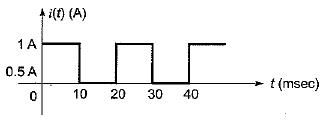Solution:

Here, time period is, T = 20 ms; between 10 ms < t < 20 ms, i(t) = 0 A.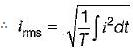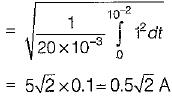Also,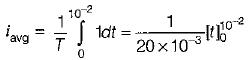= 50 x 0.01 =0.5 A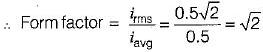QUESTION: 2

### The voltage and current in an a.c, circuit are given by v = 100 sin 314t; i = 10 sin 314t The average power in the circuit is

Solution: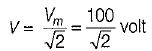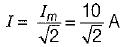∴ Pavg = IR
Now,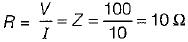(since v and i in phase)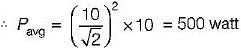QUESTION: 3

### The expression for instantaneous power dissipated in a resistor R connected across a sinusoidal alternating voltage source represented as v = Vm sin ωt is given by

Solution: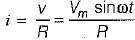∴ instantaneous power,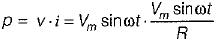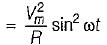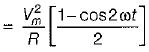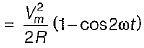QUESTION: 4

The power delivered by the two voltage sources of 6 V and 24 V as shown below will be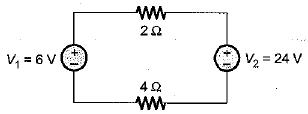Solution:

Since V1 < V2, therefore the current wil! flow from V2 to V1 as shown below.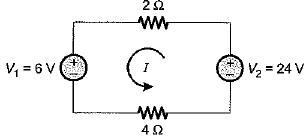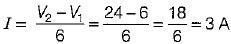Hence, the whole power in the circuit will be supplied by V2 only. Total power delivered by the voltage source V2 is
PT = I2 x (2 + 4) + 6I
= 3x 6 + 6 x 3 = 54 + 18
= 72 watts

QUESTION: 5

The power loss in watts in the resistor R shown below is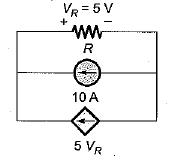Solution:

VR = (10 + 5 VR) x R
Given, VR = 5 V
So, 5 = (10 + 5 x 5) R
or,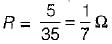∴ Power loss in R is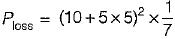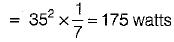QUESTION: 6

The root mean square value of the current wave given by i = (50 + 30 sin t) A will be

Solution: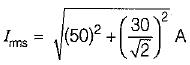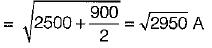= 54.30 A

QUESTION: 7

Assertion (A): In an alternating circuit, if the current is (4 - j3) amperes and the impressed voltage is (100 - j50) volts, the reactive power in the circuit is 100 VA.
Reason (R): True power is the real part of (VI).

Solution:

V = (100 - j50) volt,
I = (4 - j3) A
∴ S = VI* = (100 - j50)(4 - j3)*
= (100 - j50)(4 + j3)
= 550 + j100
∴ P = 100 W and Q = 100 VAR
Q is in VAR not in VA. Hence, assertion is not correct.
Also P is the real part of apparent power (S = VI).

QUESTION: 8

In a purely resistive circuit, the average power
Pav is______the peak power Pmax.

Solution:

Instantaneous power in a purely resistive circuit is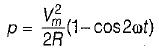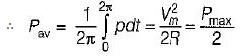QUESTION: 9

The power consumed in an inductive circuit will be

Solution:

In a purely inductive circuit, power consumed = Q = VI sin φ. In inductive circuit (having both R and L) power is consumed by resistor R which is P = VI cos φ.

QUESTION: 10

A series circuit containing passive elements has the following current and applied voltage:​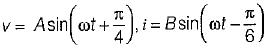The circuit elements

Solution:

Phase difference between v and i is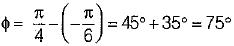∴ power factor = cos φ = cos 75° lag
Since, i lags v therefore, the circuit elements may be resistance and inductance (Z = R + jXL) or it may be resistance, inductance and capacitance Z = R + j(XL - XC), so that p.f. becomes lagging.